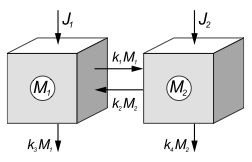Two boxes, each with inputs from and outputs to the environment, and an exchange flux between the two boxes. Adjust the parameters by dragging them, and the initial values of the boxes by changing their size.

M1

Initial value:

M1

M2

Initial value:

M2
$$\frac{\mathrm{d} M_1}{\mathrm{d} t} =$$ M1 + M2
$$\frac{\mathrm{d} M_2}{\mathrm{d} t} =$$ + M1 M2

## The formal equations

\begin{align} \frac{\mathrm{d} M_1}{\mathrm{d} t} = J_1-(k_1 + k_3)M_1 + k_2M_2\\ \frac{\mathrm{d} M_2}{\mathrm{d} t} = J_2 + k_1 M_1 - (k_2 + k_4)M_2 \end{align}